Open In App
Related Articles

# Latches in Digital Logic

Latches are digital circuits that store a single bit of information and hold its value until it is updated by new input signals. They are used in digital systems as temporary storage elements to store binary information. Latches can be implemented using various digital logic gates, such as AND, OR, NOT, NAND, and NOR gates.

### There are two types of latches:

1. S-R (Set-Reset) Latches: S-R latches are the simplest form of latches and are implemented using two inputs: S (Set) and R (Reset). The S input sets the output to 1, while the R input resets the output to 0. When both S and R are at 1, the latch is said to be in an “undefined” state.
2. D (Data) Latches: D latches are also known as transparent latches and are implemented using two inputs: D (Data) and a clock signal. The output of the latch follows the input at the D terminal as long as the clock signal is high. When the clock signal goes low, the output of the latch is stored and held until the next rising edge of the clock.
3. Latches are widely used in digital systems for various applications, including data storage, control circuits, and flip-flop circuits. They are often used in combination with other digital circuits to implement sequential circuits, such as state machines and memory elements.
4. In summary, latches are digital circuits that store a single bit of information and hold its value until it is updated by new input signals. There are two types of latches: S-R (Set-Reset) Latches and D (Data) Latches, and they are widely used in digital systems for various applications.

Latches are basic storage elements that operate with signal levels (rather than signal transitions). Latches controlled by a clock transition are flip-flops. Latches are level-sensitive devices. Latches are useful for the design of the asynchronous sequential circuit. Latches are sequential circuit with two stable states. These are sensitive to the input voltage applied and does not depend on the clock pulse. Flip flops that do not use clock pulse are referred to as latch.

SR (Set-Reset) Latch – They are also known as preset and clear states. The SR latch forms the basic building blocks of all other types of flip-flops.

SR Latch is a circuit with:
(i) 2 cross-coupled NOR gate or 2 cross-coupled NAND gate.
(ii) 2 input S for SET and R for RESET.
(iii) 2 output Q, Q’.

Under normal conditions, both the input remains 0. The following is the RS Latch with NAND gates: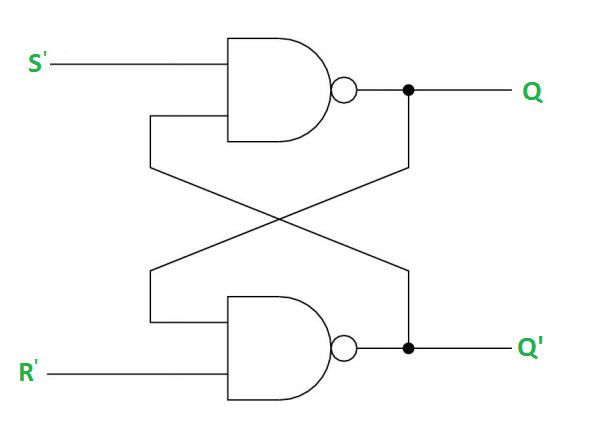Case-1: S’=R’=1 (S=R=0) –
If Q = 1, Q and R’ inputs for 2nd NAND gate are both 1.
If Q = 0, Q and R’ inputs for 2nd NAND gate are 0 and 1 respectively.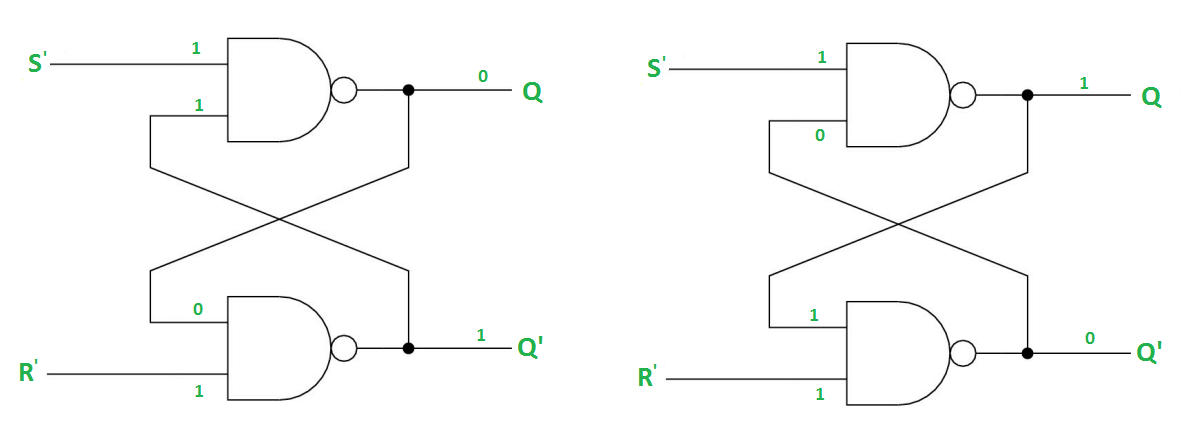Case-2: S’=0, R’=1 (S=1, R=0) –
As S’=0, the output of 1st NAND gate, Q = 1(SET state). In 2nd NAND gate, as Q and R’ inputs are 1, Q’=0.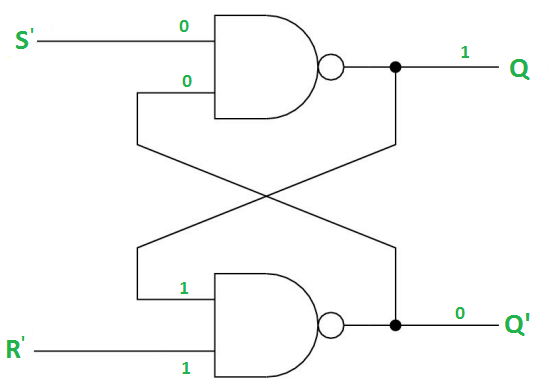Case-3: S’= 1, R’= 0 (S=0, R=1) –
As R’=0, the output of 2nd NAND gate, Q’ = 1. In 1st NAND gate, as Q and S’ inputs are 1, Q=0(RESET state).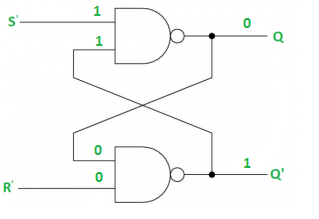Case-4: S’= R’= 0 (S=R=1) –
When S=R=1, both Q and Q’ becomes 1 which is not allowed. So, the input condition is prohibited.

The SR Latch using NOR gate is shown below: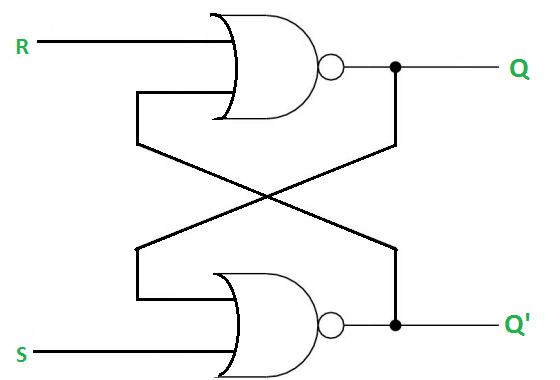Gated SR Latch –
A Gated SR latch is a SR latch with enable input which works when enable is 1 and retain the previous state when enable is 0.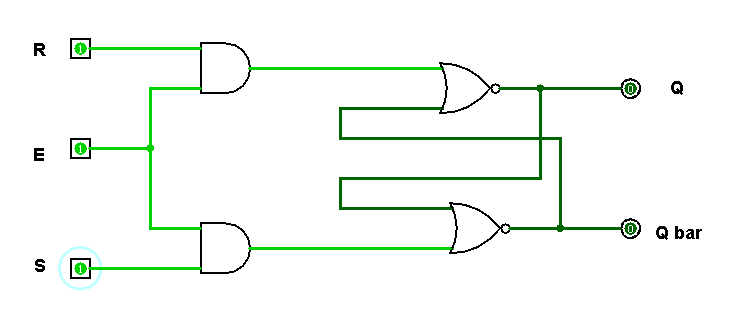Gated D Latch –
D latch is similar to SR latch with some modifications made. Here, the inputs are complements of each other. The letter  in the D latch stands for “data” as this latch stores single bit temporarily.

The design of D latch with Enable signal is given below: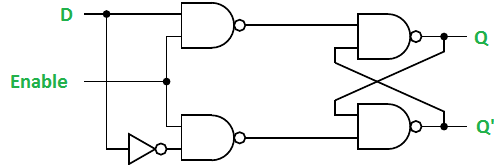The truth table for the D-Latch is shown below:

As the output is same as the input D, D latch is also called as Transparent Latch. Considering the truth table, the characteristic equation for D latch with enable input can be given as:

`Q(n+1) = EN.D + EN'.Q(n)`

1. Easy to Implement: Latches are simple digital circuits that can be easily implemented using basic digital logic gates.
2. Low Power Consumption: Latches consume less power compared to other sequential circuits such as flip-flops.
3. High Speed: Latches can operate at high speeds, making them suitable for use in high-speed digital systems.
4. Low Cost: Latches are inexpensive to manufacture and can be used in low-cost digital systems.
5. Versatility: Latches can be used for various applications, such as data storage, control circuits, and flip-flop circuits.

1. No Clock: Latches do not have a clock signal to synchronize their operations, making their behavior unpredictable.
2. Unstable State: Latches can sometimes enter into an unstable state when both inputs are at 1. This can result in unexpected behavior in the digital system.
3. Complex Timing: The timing of latches can be complex and difficult to specify, making them less suitable for real-time control applications.

### Reference:

Here are a few books that you can refer to for further information on latches:

1. “Digital Design: Principles and Practices” by John F. Wakerly
2. “Digital Systems Design using VHDL” by Charles H. Roth and Lizy Kurian John
3. “Digital Circuit Analysis and Design” by Victor P. Nelson and H. Troy Nagle
4. “Digital Design and Computer Architecture” by David Harris and Sarah Harris
5. “Fundamentals of Digital Logic with Verilog Design” by Stephen Brown and Zvonko Vranesic

These books provide a comprehensive overview of digital logic, including latches, and cover various topics, such as design and implementation, simulation, and verification of digital circuits.

DIGITAL ELECTRONICS – Atul P. Godse, Mrs. Deepali A. Godse